# SAT Math Multiple Choice Question 955: Answer and Explanation

### Test Information

Question: 955

7.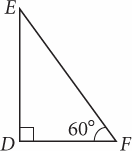The area of triangle DEF is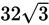square units. What is the length of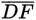?

• A.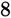• B.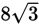• C.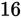• D.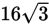Explanation:

A

Difficulty: Medium

Category: Additional Topics in Math / Geometry

Strategic Advice: When you see a triangle on Test Day that has a 60-degree angle, check the formula page at the beginning of the math section and jot down the ratio for the sides of a 30-60-90 triangle,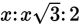.

Getting to the Answer: You're given the area of the triangle. Because the triangle is a right triangle, the base and the height are the two legs of the triangle. Call the lengths of the legs x and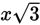. Substitute these values and the given area into the area formula: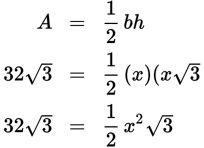To solve this equation, divide both sides by the radical, and then multiply both sides by 2.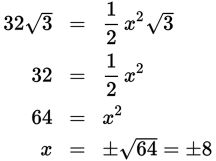Length can't be negative, so x = 8, which is the length of the shortest leg. Sideis across from the 30-degree angle (which is the smallest angle in the triangle), so it is the shortest leg (which is given by x), making (A) correct.